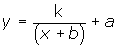Learn from home

The teachersThe criterion is given by a quotient between polynomials:The domain is equal to, minus the values of x that would annul the denominator.

The functions of the typehas a hyperbola in its graph.

Also, hyperbolas are the graphs of the functions.

The simplest hyperbola is represented with the equation.

Its asymptotes are the axes.

The center of the hyperbola, which is where the asymptotes intersect, is the origin.## 1. Vertical TranslationThe center of the hyperbola is (0, a).

If a>0,moves upward a units.The center of the hyperbola is: (0, 3)

If a<0,moves down a units.The center of the hyperbola is: (0, −3)

## 2. Horizontal TranslationThe center of the hyperbola is: (−b, 0).

If b> 0,is shifted to the left b units.The center of the hyperbola is: (−3, 0)

If b<0,is shifted to the right b units.The center of the hyperbola is: (3, 0)

## 3. Oblique TranslationThe center of the hyperbola is: (−b, a).The center of the hyperbola is: (3, 4).

To graph hyperbolas of the type:It is divided and is written as:Its graph is a hyperbola with a center (−b, a) and asymptotes parallel to the axes.The center of the hyperbola is: (−1, 3).

Did you like the article?(1 votes, average: 5.00 out of 5)Loading...

Emma

I am passionate about travelling and currently live and work in Paris. I like to spend my time reading, gardening, running, learning languages and exploring new places.

Did you like
this resource?

Bravo!